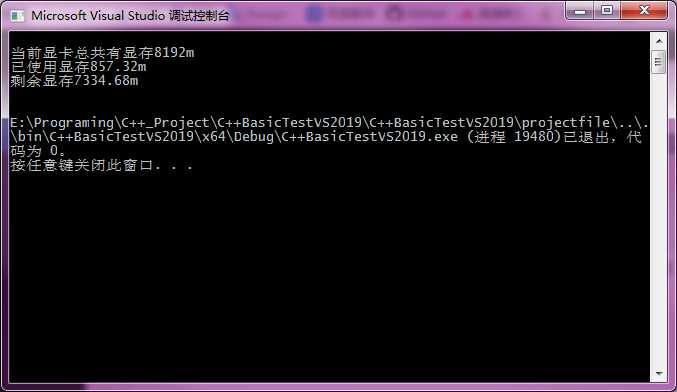# 1 C++使用cuda api获取当前GPU显卡的显存容量、已使用显存、剩余显存

#include <iostream>
#include "cuda_runtime_api.h"

static void GetGPUMemory()
{
int deviceCount = 0;
cudaError_t error_id = cudaGetDeviceCount(&deviceCount);
if (deviceCount == 0)
{
std::cout << "当前PC没有支持CUDA的显卡硬件设备" << std::endl;
}

size_t gpu_total_size;
size_t gpu_free_size;

cudaError_t cuda_status = cudaMemGetInfo(&gpu_free_size, &gpu_total_size);

if (cudaSuccess != cuda_status)
{
std::cout << "Error: cudaMemGetInfo fails : " << cudaGetErrorString(cuda_status) << std::endl;
exit(1);
}

double total_memory = double(gpu_total_size) / (1024.0 * 1024.0);
double free_memory = double(gpu_free_size) / (1024.0 * 1024.0);
double used_memory = total_memory - free_memory;

std::cout << "\n"
<< "当前显卡总共有显存" << total_memory << "m \n"
<< "已使用显存" << used_memory << "m \n"
<< "剩余显存" << free_memory << "m \n" << std::endl;

}

int main()
{
GetGPUMemory();
return 0;
}